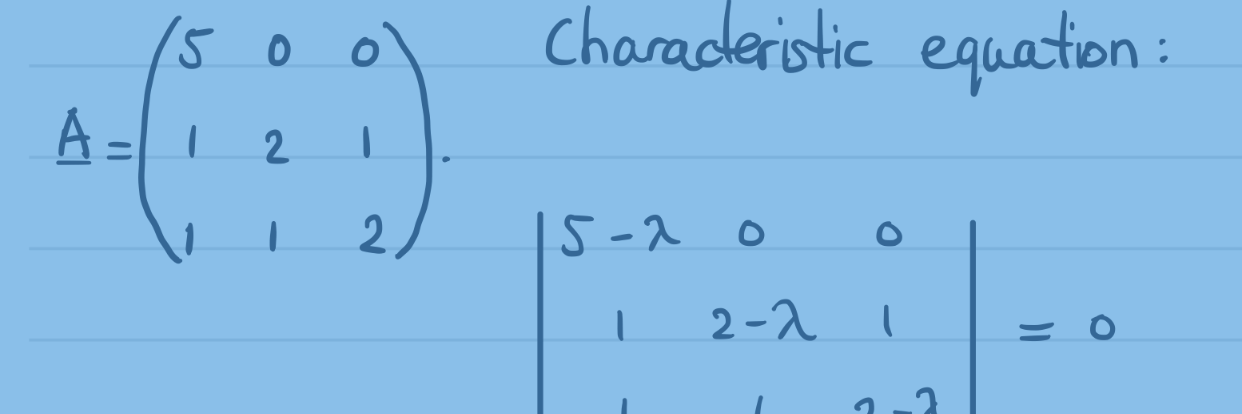# Real eigenvalues and eigenvectors of 3×3 matrices, example 3

We calculate the eigenvalues and eigenvectors of a 3×3 matrix in real number space. Continue reading Real eigenvalues and eigenvectors of 3×3 matrices, example 3# Real eigenvalues and eigenvectors of 3×3 matrices, example 2

We calculate the eigenvalues and eigenvectors of a 3×3 matrix in real number space. Continue reading Real eigenvalues and eigenvectors of 3×3 matrices, example 2# Real eigenvalues and eigenvectors of 3×3 matrices, example 1

We calculate the eigenvalues and eigenvectors of a 3×3 matrix in real number space. Continue reading Real eigenvalues and eigenvectors of 3×3 matrices, example 1